# Creating a Normal Star in Adobe Illustrator, American Flag Style

by

12/2/2012 Update: The normal star effect can be achieved by holding down the alt/option key when creating the star via click-and-drag.

With the Star Tool in Adobe Illustrator, the shape of a star can be modified by specifying the number of star points, an outer radius, and an inner radius. Illustrator’s default 5-point star is pudgy; to make a normal star like the one on the American flag, Radius 1 and Radius 2 must be in such a proportion that the edges of each point are visually in line with the edges of the points across the star.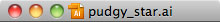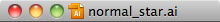## Formula for Calculating Radius

``````inner_radius = outer_radius * .382
``````

For a star with an outer radius of 100, the inner radius would be 38.2. The dialog to specify these values appears when you select the Star Tool and click (not click-and-drag).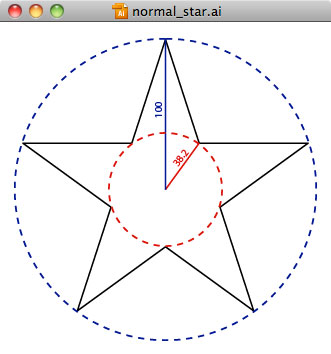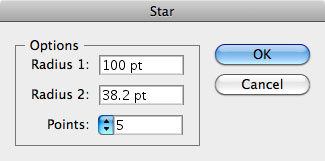## Proof

To calculate the proportion of Radius 2 to Radius 1, I sought to determine the 3 angles of the gray triangle below so I could then determine the proportion of the sides: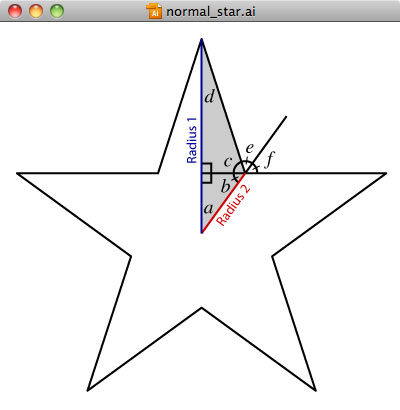• to start, we know that angle a is 36° because it is one tenth of a 360° circle (one half of one fifth)
• for American stars, non-adjacent star points can be connected with a line, so we can draw out this line to treat the gray triangle as two right triangles
• we know two of the angles in the bottom right triangle, so angle b must be 54°
• angle f is opposite angle b, so it is also 54° as is angle e
• angle c is 72° since it is supplementary with the combination of angle e and angle f.
• angle d = 180° - angle a - angle b - angle c = 18°
• finally, now that we know the all the angles of the gray triangle, we can use trigonometry to determine that if Radius 1 is 100, Radius 2 would be 38.2